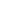Share

# Floating Point

As the name implies, floating point numbers are numbers that contain floating decimal points. For example, the numbers 5.5, 0.001, and -2,345.6789 are floating point numbers. Numbers that do not have decimal places are called integers.

Computers recognize real numbers that contain fractions as floating point numbers. When a calculation includes a floating point number, it is called a "floating point calculation." Older computers used to have a separate floating point unit (FPU) that handled these calculations, but now the FPU is typically built into the computer's CPU.

Published: 2007

Definition from the PC Glossary
https://pc.net/glossary/floating_point